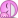# Dx before the f(x) in integrals

Demystifier
Gold Member
•fresh_42
stevendaryl
Staff Emeritus
Which doesn't say anything about the notation ##\int fdx##.
But it does seem to write the Lebesgue integral in terms of infinitesimals.

But it does seem to write the Lebesgue integral in terms of infinitesimals.
But those infinitesimals are for another function. The point was to be able to make sense,at least intuitively, of ##\int dxf(x)## as an infinite sum of infinitesimals.

Demystifier
Gold Member
Would it be correct to say that both Riemann and Lebesque integrals are just special cases of computing the area
$$\int\int dxdy$$
of a region on a 2-dimensional plane?

And what do you mean by area? The Lebesgue measure of the set? The integral you wrote down? What exactly is that integral?

stevendaryl
Staff Emeritus
And what do you mean by area? The Lebesgue measure of the set? The integral you wrote down? What exactly is that integral?
Let ##f## be a function from reals to reals (for simplicity, let it be nonnegative). Let ##S(A,B)## be the set of points ##(x,y)## such that ##A \leq x \leq B## and ##0 \leq y \leq f(x)##. Then if the area of set ##S(A,B)## is defined, it will be equal to ##\int_A^B f(x) dx##. Right? By "area" I mean a measure on ##R^2## such that every set of the form ##\{ (x,y) | x_0 \leq x \leq x_1 \wedge y_0 \leq y \leq y_1\}## has measure ##|(x_1 - x_0) \cdot (y_1 - y_0)|##

Let ##f## be a function from reals to reals (for simplicity, let it be nonnegative). Let ##S(A,B)## be the set of points ##(x,y)## such that ##A \leq x \leq B## and ##0 \leq y \leq f(x)##. Then if the area of set ##S(A,B)## is defined, it will be equal to ##\int_A^B f(x) dx##. Right? By "area" I mean a measure on ##R^2## such that every set of the form ##\{ (x,y) | x_0 \leq x \leq x_1 \wedge y_0 \leq y \leq y_1\}## has measure ##|(x_1 - x_0) \cdot (y_1 - y_0)|##
May be, it depends on the unstated assumptions. Say you take area to mean the Lebesgue measure in the plane. It satisfies your requirement for area. Take a function ##f(x)## so that ##S(A,B)## is Lebesgue measurable and ##f(x)## is not Riemann integrable. Then if the integral you wrote is the Riemann integral, then the area is not it, the integral doesn't exists. I assume that is not what you mean, but you didn't say anything about the relationship between the measure in the plane and the integral on the line in you statement. But let's say that it is all fine. Then what? Where are you going with this?

stevendaryl
Staff Emeritus
But let's say that it is all fine. Then what? Where are you going with this?
I was just trying to answer the question "What do you mean by area"?

##\int dx f(x) = (x+C)f(x) ##Would it be correct to say that both Riemann and Lebesque integrals are just special cases of computing the area
$$\int\int dxdy$$
of a region on a 2-dimensional plane?
I'm having difficulty in understanding how either of them would be special cases of finding an 'area'. An application of either theory would be finding the area/volume/measure of an ##n##-dimensional, pre-determined to be measurable, set.

The expression ##\int\int dx\,dy ## is just some function ##f:\mathbb R ^2\to\mathbb R## (hopefully).

Last edited: